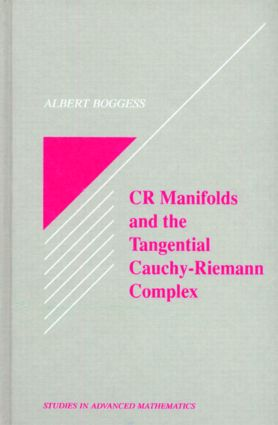CR Manifolds and the Tangential Cauchy Riemann Complex

1st Edition

CRC Press

384 pages

Purchasing Options:\$ = USD
Hardback: 9780849371523
pub: 1991-09-12
SAVE ~\$48.00
\$240.00
\$192.00
x
eBook (VitalSource) : 9781315140445
pub: 2017-09-20
from \$28.98

FREE Standard Shipping!

Description

CR Manifolds and the Tangential Cauchy Riemann Complex provides an elementary introduction to CR manifolds and the tangential Cauchy-Riemann Complex and presents some of the most important recent developments in the field. The first half of the book covers the basic definitions and background material concerning CR manifolds, CR functions, the tangential Cauchy-Riemann Complex and the Levi form.

The second half of the book is devoted to two significant areas of current research. The first area is the holomorphic extension of CR functions. Both the analytic disc approach and the Fourier transform approach to this problem are presented. The second area of research is the integral kernal approach to the solvability of the tangential Cauchy-Riemann Complex. CR Manifolds and the Tangential Cauchy Riemann Complex will interest students and researchers in the field of several complex variable and partial differential equations.

PRELIMINARIES. Differential Forms and Stokes Theorem. Distributions and Curents. Fundamental Solutions for(these symbols are to be used in an equation -----? ? z ---- see note at bottom)and D. Edge of the Wedge Theorem. Whitney's Extension Theorem. CR MANIFOLDS AND CR FUNCTIONS. Overview. CR Manifolds. The Tangential Cauchy Riemann Complex. Normal Form for Imbedded CR Manifolds. CR Functions. The Imbeddability of Real Analytic Abstract CR Manifolds. A Non-Imbeddable C8 Abstract CR Manifold. Further Results. HOLOMORPHIC EXTENSION OF CR FUNCTIONS. Overview. Approximating CR Functions By Entire Functions. Statement of the Extension Theorem. The Analytic Disc Technique. The Fourier Transform Technique. The Real Analytic Regularity of CR Mappings. Further Results. THE SOLVABILITY OF THE TANGENTIAL CAUCHY RIEMANN EQUATIONS. Overview. Kernel Calculus. The Kernels of Cauchy and Bochner. The Kernel of Henkin. The Fundamental Solution for the Tangential Cauchy Riemann Equations. A Local Solution for the Tangential Cauchy Riemann Equations. Lewy's Non-Solvability Example. Henkin's Criterion for Local Solvability of the Tangential Cauchy Riemann Complex at the Top Degree. Further Results.